# 非均匀复变函数的级数理论Series Theory of Heterogeneous Complex Variable Function

• 全文下载: PDF(399KB)    PP.178-186   DOI: 10.12677/AAM.2020.92021
• 下载量: 62  浏览量: 116   科研立项经费支持

In this paper, the series theory of complex variable function is applied to heterogeneous complex variable function. The convergence region and the radius of convergence ellipse of heterogeneous power series are established by using the theory of heterogeneous analytic function. At the same time, the Taylor’s theorem of heterogeneous analytic function is established by using the hetero-geneous Cauchy integral formula and the high order derivative formula of Cauchy integral.

1. 引言

2. 预备知识

2.1. 非均匀复数的定义

${C}_{K}$ 中引入数乘

$z=a+jb,z\in {C}_{K},m\in R,mz=ma+jmb,$

${C}_{K}$ 中引入加法

${z}_{1}={a}_{1}+j{b}_{1},{z}_{2}={a}_{2}+j{b}_{2},{z}_{1}\in {C}_{K},{z}_{2}\in {C}_{K},$

${z}_{1}+{z}_{2}=\left({a}_{1}+{a}_{2}\right)+j\left({b}_{1}+{b}_{2}\right).$

2.2. 非均匀复变函数的定义及性质

$\frac{\Delta w}{\Delta z}=\frac{f\left(z\right)-f\left({z}_{0}\right)}{z-{z}_{0}}=\frac{f\left({z}_{0}+\Delta z\right)-f\left({z}_{0}\right)}{\Delta z}\text{ }\text{ }\text{ }\text{ }\text{ }\text{ }\text{ }\text{ }\text{ }\text{ }\text{ }\text{ }\text{ }\text{ }\text{ }\left(\Delta z\ne 0\right)$

${f}^{\prime }\left({z}_{0}\right)=\underset{\Delta z\to 0}{\mathrm{lim}}\frac{\Delta w}{\Delta z}=\underset{\Delta z\to 0}{\mathrm{lim}}\frac{f\left(z\right)-f\left({z}_{0}\right)}{z-{z}_{0}},$

1) 偏微分 ${p}_{x},{p}_{y},{q}_{x},{q}_{y}$ 在区域D内连续；

2) $p\left(x,y\right),q\left(x,y\right)$ 在区域D内满足非均匀C.-R.方程。

2.3. 非均匀复变函数的积分

$z=z\left(t\right)\text{}\left(\alpha \le t\le \beta \right)$

$a=z\left(\alpha \right)$ 为起点， $b=z\left(\beta \right)$ 为终点， $f\left(z\right)$ 沿C有定义，顺着C从a到b的方向在C上取分点： $a={z}_{0},{z}_{1},\cdots ,{z}_{n-1},{z}_{n}=b$，这样可以将曲线C划分为n个弧段，在从 ${z}_{t-1}$${z}_{t}$ 的每一个弧段上任取一点 ${\xi }_{t}$，那么

${S}_{n}=\underset{t=1}{\overset{n}{\sum }}f\left({\xi }_{t}\right)\Delta {z}_{t},\text{}\Delta {z}_{t}={z}_{t}-{z}_{t-1}$

${\int }_{C}f\left(z\right)\text{d}z={\int }_{C}u\text{d}x-kv\text{d}y+j{\int }_{C}v\text{d}x+u\text{d}y.$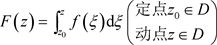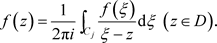3. 非均匀复级数理论文

3.1. 非均匀复数项级数基本性质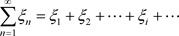(3.1)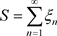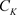是非均匀复数域，在上定义非均匀模若满足下面的关系，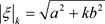，其中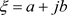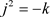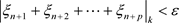3.2. 一致非均匀收敛的非均匀复函数项级数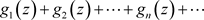(3.2)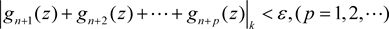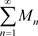收敛，则非均匀复函数项级数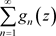在非均匀复数域的集合E上绝对收敛且一致收敛：我们称级数为非均匀复函数项级数的优级数。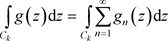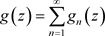2)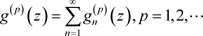2) 利用高阶导数的非均匀Cauchy积分公式(文献  定理4.8)和等式，结论是显然的。

3.3. 非均匀幂级数敛散性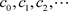和a是非均匀复常数，我们称下面的级数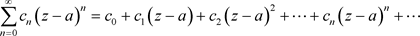(3.3)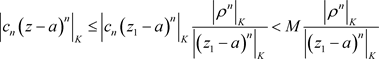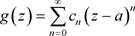(3.4)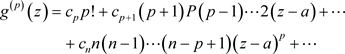(3.5)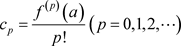。 (3.6)

1)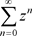的收敛椭圆半径为1，且，当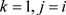，与复变函数的理论一致，且收敛到解析函数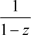，当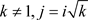，转化成复数项级数的形式，利用复变函数级数理论，非均匀幂级数内绝对且内闭一致收敛于非均匀解析函数2)的收敛半径为，且如1)一样的方法可知：非均匀幂级数在收敛椭圆域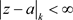内绝对且内闭一致收敛于非均匀解析函数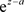3)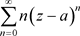的收敛半径为0，因此除处处发散。

3.4. 非均匀解析函数的泰勒级数展开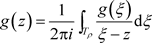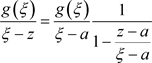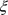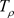上时，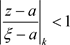，由例子3.17，1)李忠. 复分析导引[M]. 北京: 北京大学出版社, 2004.  钟玉泉. 复变函数论[M]. 北京: 高等教育出版社, 2012.  戴滨林, 杨世海. 复变函数[M]. 上海: 复旦大学出版社, 2019.  赵雪娇, 陈雲, 陶继成. 非均匀复数和非均匀复变函数[J]. 应用数学进展, 2017, 6(1): 69-77.  陈雲, 赵雪娇, 陶继成. 非均匀复变函数的积分[J]. 应用数学进展, 2017, 6(2): 153-164.  Tao, J.C., Chen, Y. and Zhao, X.J. (2017) Heterogeneous Complex Function. 2017 International Conference on Applied Mathematics, Modelling and Statistics Application, Beijing, China, May 22-27 2017, 345-348. https://doi.org/10.2991/ammsa-17.2017.77